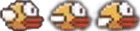# ShymeanV0.8.0

<!--more-->

## 1. 逐帧动画

• 提前做好每一帧的静态图片，为了减少网络请求，一般会使用精灵图，然后通过`background-postion`来控制动画的展示
• 按顺序播放每一帧，形成动画效果

CSS可以通过`@keyframes`来定义关键帧动画，每个关键帧有一个百分比值作为名称，代表在动画进行中，在哪个阶段触发这个帧所包含的样式。

• cubic-bezier() 定时函数
• steps() 函数

1. 准备下面这张模糊到不行的精灵图2. 定义三帧对应的css属性，主要是找到每一帧的背景偏移量

``````.bird {
position: relative;
width: 46px;
height: 31px;
background-image: url("./bird.png");
background-repeat: no-repeat;
}
.bird1 {
background-position: 0 center;
}
.bird2 {
background-position: -47px center;
}
.bird3 {
background-position: -94px center;
}``````
3. 把上面的三帧放在动画中

``````@keyframes fly-bird {
0% {
background-position: 0;
}

33.3333% {
background-position: -47px;
}

66.6666% {
background-position: -94px;
}
}``````
4. 运行动画

``````.bird-fly {
animation: fly-bird 0.4s steps(1) infinite;
}``````

``````@keyframes fly-bird2 {
100% {
background-position: -141px;
}
}

.bird-fly {
// 指定3帧，由step自己计算每一帧的偏移
animation: fly-bird2 0.4s steps(3, end) infinite;
}``````

## 2. SVG描边动画

SVG描边动画时SVG中最常用的一种动画形式，其核心原理是与两个属性有关

• stroke-dasharray，主要用来绘制虚线，其值为`x,y`的形式，其中x表示实线的长度dash，y表示两根实线之间的间隔gap
• stroke-dashoffset，用来定义dash线条开始的位置

path的路径并不一定是规则的，所幸的是JavaScript提供了获取path路径长度的接口

``````let path = document.querySelector('path');
let length = path.getTotalLength(); // 将这个值作为dashoffset和dasharray的取值``````

``````@keyframes stroke {
100% {
stroke-dashoffset: 0;
}
}

.chat_corner {
animation: stroke 0.6s linear 0.3s forwards;
}

.chat-path {
width: 110px;
margin: -5px auto 0;
}

.chat-path .chat_corner {
transform-origin: 50% 50%;
stroke-dasharray: 641;
stroke-dashoffset: 641;
}``````

## 3. canvas动画

canvas动画可以理解成JS版的逐帧动画，主要原理是在每一帧手动计算元素的状态并将其绘制到画布上。

### 3.1. 基本动画

``````let canvas = document.getElementById("myCanvas");
let ctx = canvas.getContext("2d");

let ball = {
x: 0,
y: 100,
r: 10,
dx: 2,
rgbaColorArr: [111, 123, 222, 1],
draw() {
const {x, y, r, rgbaColorArr} = this
ctx.beginPath()
ctx.arc(x, y, r, 0, Math.PI * 2);
ctx.closePath()
ctx.fillStyle = `rgba(\${rgbaColorArr.join(',')})`
ctx.fill();
},
move() {
this.x += this.dx
this.draw()
},
animate() {
const d = 1000 // 动画时长 1000ms
const update = (t) => {
// 清除画布
ctx.clearRect(0, 0, canvas.width, canvas.height);
// 移动小球并重新绘制
this.x += this.dx
this.draw()

if (t < d) {
requestAnimationFrame(update);
}
}
requestAnimationFrame(update);
}
}

ball.animate()``````

• 通过`requestAnimationFrame`清除画布并绘制每一帧的内容
• 在每一帧中，修改`this.x`横坐标的位置，然后调用`ball.braw`方法重新绘制小球

``this.x += this.dx``

• 第一帧小球的横坐标为`x + dx`
• 第二帧小球的横坐标为`x + 2*dx`
• ...

``````// t: 当前时间
// b: 开始的状态
// c: 变化的状态
// d: 从b变成b+c需要的时间
function linear(t, b, c, d) {
return c * t / d + b
}
function easeIn(t, b, c, d) {
return c * (t /= d) * t + b;
}``````

``````let ball = {
// ... 其他属性
animate() {
const d = 1000 // 运动时间1000ms
const target = canvas.width - this.r * 2 // 目标位置

const update = (t) => {
ctx.clearRect(0, 0, canvas.width, canvas.height);
// this.x += this.dx
// this.x = linear(t, 0, target, d) 等价于上面的this.x += this.dx
this.x = easeIn(t, 0, target, d) // 缓动函数easeIn
this.draw()

if (t < d) {
requestAnimationFrame(update);
}
}
requestAnimationFrame(update);
}
}``````

### 3.2. 粒子动画

• 定义粒子，一个粒子的基本属性包括：位置、尺寸、颜色、速度、停留时长等
• 生成粒子，可以直接遍历动态生成，也可以提前准备好粒子（如使用`getImageData`获取画布上某个区域的像素点)
• 发射粒子，将粒子渲染在每一帧的画布上，这一步跟上面单个小球的动画展示很像，只是我们现在需要处理成千上万个粒子

#### 3.2.1. 基础效果

``````const pos = {x: 50, y: 50, w: 100, h: 100}
const originX = pos.x + pos.w / 2
const originY = pos.y + pos.h + 200

// 使用一个数组保存生成的粒子
let particles = []
const reductionFactor = 5
for (let x = 0; x < pos.w; x += reductionFactor) {
for (let y = 0; y < pos.h; y += reductionFactor) {
let particle = {
// 初始位置
x0: originX,
y0: originY,
// 终止位置
x1: x + pos.x,
y1: y + pos.y,
// 样式属性
rgbaColorArr: randomColor(),
currTime: 0, // 粒子已经运行的时间
duration: 3000,
};
particles.push(particle);
}
}``````

``````function easeInOutExpo(e, a, g, f) {
return g * (-Math.pow(2, -10 * e / f) + 1) + a
}

let requestId

function renderParticles(t) {
// 最后一个粒子
const last = particles[particles.length - 1]
if (last.duration < last.currTime) {
cancelAnimationFrame(requestId)
return
}

ctx.clearRect(0, 0, canvas.width, canvas.height);

for (let p of particles) {
const {duration, currTime} = p
ctx.fillStyle = `rgba(\${p.rgbaColorArr.join(',')})`
if (currTime < duration) {
let x = easeInOutExpo(currTime, p.x0, p.x1 - p.x0, duration);
let y = easeInOutExpo(currTime, p.y0, p.y1 - p.y0, duration);
ctx.fillRect(x, y, 1, 1)

} else {
ctx.fillRect(p.x1, p.y1, 1, 1)
}
p.currTime = t
}

requestId = requestAnimationFrame(renderParticles)
}

const animate = () => {
requestId = requestAnimationFrame(renderParticles)
}

animate()``````

#### 3.2.2. 让粒子动画更真实

• 让每一行粒子启动时间有规律错开
• 每一行粒子之间启动时间随机的错开

``````const frameTime = 16.66 // 假设一帧为16.66ms

for (let x = 0; x < pos.w; x += reductionFactor) {
for (let y = 0; y < pos.h; y += reductionFactor) {
let particle = {
// ... 其他参数
delay: y / 20 * frameTime, // 延迟启动的时间，按行来区分，突出层次感
interval: parseInt(Math.random() * 10 * frameTime), // 每个粒子的启动间隔时间，随机1到10帧，突出粒子的颗粒感
}
particles.push(particle);
}
}``````

``````for (let p of particles) {
// 未到启动时间，则直接跳过
if (p.currTime < p.delay) {
p.currTime = t
continue
}

const {duration, currTime, interval} = p
ctx.fillStyle = `rgba(\${p.rgbaColorArr.join(',')})`
if (currTime < duration + interval) {
// 引入interval控制每个粒子的启动间隔
if (currTime >= interval) {
let x = easeInOutExpo(currTime - interval, p.x0, p.x1 - p.x0, duration)
let y = easeInOutExpo(currTime - interval, p.y0, p.y1 - p.y0, duration)
ctx.fillRect(x, y, 1, 1)
}
} else {
ctx.fillRect(p.x1, p.y1, 1, 1)
}
p.currTime = t
}``````

## 4. FLIP实现DOM切换动画

FLIP stands for First, Last, Invert, Play.

FLIP是一种实现DOM状态切换动画的方案

• First: 元素的初始状态
• Last: 元素的终止状态
• Invert: 这是整个动画实现的核心思想，比如一个元素初始x位置是0，向右移动到了90px的位置，为了实现动画效果，我们可以将元素的初始状态设置成`transform: translate`
• Play: 播放动画，即动画回到他本来的位置

``````function getPositionList(domList) {
return domList.map((dom) => {
const {left, top} = dom.getBoundingClientRect()
return {left, top}
})
}

this.list = shuffle(this.list)

const cellList = this.\$refs.cell.slice() // 保存快照

// 获取初始状态
const originPositions = getPositionList(cellList)

this.\$nextTick(() => {
// 获取节点新的状态
// 需要保证变化前后的DOM节点一致，因此v-for的key不能使用index
const currentPositions = getPositionList(cellList)

cellList.forEach((cell, index) => {
let cur = currentPositions[index]
let origin = originPositions[index]

const invert = {
left: origin.left - cur.left,
top: origin.top - cur.top,
}

const keyframes = [
{transform: `translate(\${invert.left}px, \${invert.top}px)`},
{transform: "translate(0)"},
]

const options = {
duration: 300,
easing: "linear",
}

cell.animate(keyframes, options)
})
})``````

## 5. lottie：抱住设计大腿

Lottie是基于CALayer的动画, 所有的路径预先在AE中计算好, 转换为Json文件, 然后自动转换为Layer的动画,

• 导出`lottie-web`库，导入动画JSON文件
• `lottie.loadAnimation`运行文件

lottie可以运行在iOS、Android甚至于Flutter等多个平台，当需要一些比较复杂的动画时，去求求设计大佬吧

## 6. 小结

• 逐帧动画，最常见的动画形式
• 描边动画，使用SVG实现的path动画效果
• 粒子动画，使用canvas实现酷炫的粒子效果
• FLIP动画，主要用于DOM切换等效果